﻿ Heating due to the Iron Loss (AC Analysis with B-H Curve Taken into Account)Examples | Product | Murata Software Co., Ltd.# Example2Heating due to the Iron Loss (AC Analysis with B-H Curve Taken into Account)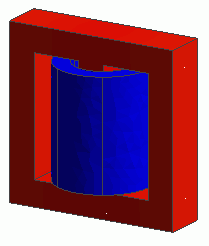General

• A coil is wound on a core. The heating due to the iron loss is analyzed.

• Permeability is set with B-H curve table.
The effect of magnetic saturation is taken into account in this exercise whereas it cannot be taken into account in the [Exercise 3: Heating due to the Iron Loss] of magnetic field harmonic analysis.

• The vectors of the magnetic field, the loss in the core, and the temperature distribution by the heating are solved.

• Unless specified in the list below, the default conditions will be applied.

### Analysis Space

 Item Setting Analysis Space 3D Model unit mm

### Analysis Conditions

 Item Setting Solver Magnetic Field Analysis [Luvens] Thermal Analysis [Watt] Analysis Type Magnetic field analysis: Transient Analysis Thermal analysis: Steady-State Analysis Options None

The Transient Analysis tab is set up as follows.

50KHz AC is input. To reproduce its waveform well, the time step is set by dividing the periodic time of 50KHz by 12.

Tab

Setting Item

Setting

Transient Analysis

Time step

Automatic

Table

 Number Calculation steps Output steps Time step [s] 1 12 1 1.0/(12*50e3)

### Graphical Objects

A coil is wound on a core.

The bodies of a core (Core) and a loop coil (Coil) are defined.

The automatically created ambient air is applicable only in the magnetic field analysis, not in the thermal analysis.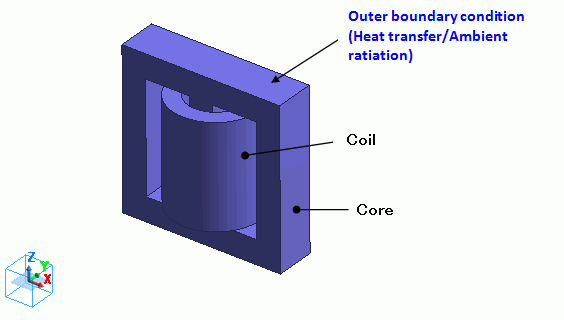### Body Attributes and Materials

 Body Number/Type Body Attribute Name Material Name 5/Solid Coil2 008_Cu * 7/Solid Coil1 008_Cu * 6/Solid Core Core

(*) Available from the Material DB

Body attribute is set up as follows to apply current to the loop coil.

 Body Attribute Name Tab Setting Coil Current Waveform: AC Current: 0.1[A] Turns: 100[Turns] Induced current: None Direction: Loop Coil/Magnetic Field Direction Direction Vector of Magnetic Field: X=0, Y=0, Z=1

The material properties of the core are set up as follows. The loss is defined in the iron loss table.

Material Name

Tab

Setting

Core

Permeability

Magnetization Characteristic Type: Select B-H curve

B-H Curve Table

 Magnetic Field [A/m] Magnetic Flux Density [T] 0 0 58 0.219 90 0.33 180 0.59 380 0.69 1100 0.75 2000 0.78 3000 0.8 11000 0.9 20000 0.95

Electric Conductivity

Conductivity Type: Conductor

Conductivity: 0.1[S/m]

Thermal Conductivity

Thermal Conductivity: 10[W/m/deg]

Iron Loss

Iron Loss Calculation Type: Iron loss table

Frequency: 5×104[Hz]

[Magnetic flux density-Loss density] Table *

 Magnetic Flux Density [T] Joule Loss Density [W/m^3] 50×10-3 5×10^3 500×10-3 500×10^3

* This is not the actual material’s property.

Press the Graph button. The following graph will show up.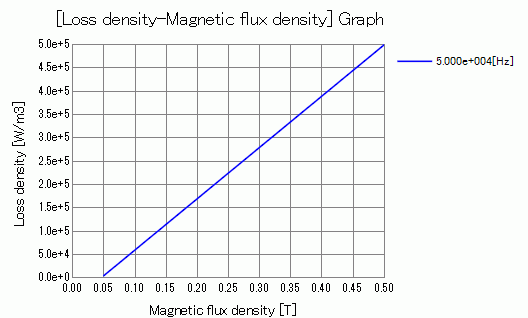### Boundary Condition

“Natural convection (automatic calculation)” is set on the outer boundary condition (surrounding the coil and core in the case of the thermal analysis).

 Boundary Condition Name/Topology Tab Boundary Condition Type Setting Outer Boundary Condition * Thermal Heat Transfer/Ambient Radiation Natural convection (automatic calculation) Room temperature: 25[deg]

To set Outer Boundary Condition, go to the [Model] tab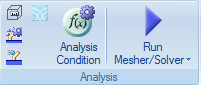and click [Outer Boundary Condition]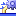.

* The correction coefficient for the natural convection is calculated automatically. See [Heat Transfer/Ambient Radiation] for more information.

To see the iron loss, go to the [Results] taband click [Table]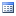.

The joule loss is shown below.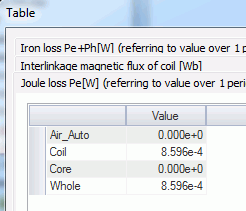The iron loss is shown below.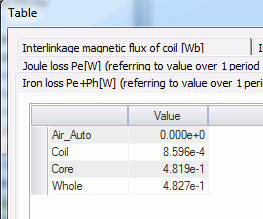The core’s iron loss is approximately 0.5[W].

The joule loss and the hysteresis loss of the core are not given because the the material property is defined on the iron loss table.

The loss characteristics are defined in the iron loss table. Only iron loss is obtained.

If it is defined by the iron loss experimental formula, the joule loss and the hysteresis loss will be output as well.

See [Loss Calculation in the Magnetic Field Analysis] for details of the loss.

The vectors of the magnetic field are shown below.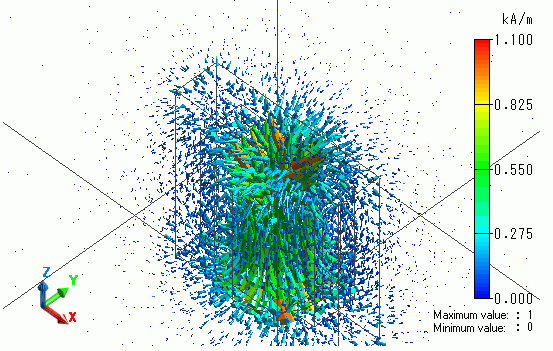The vectors of the magnetic flux density are shown below.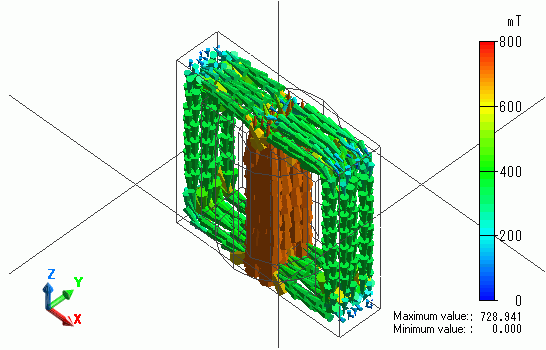The flux density is looping through the core.

The iron loss density is shown below.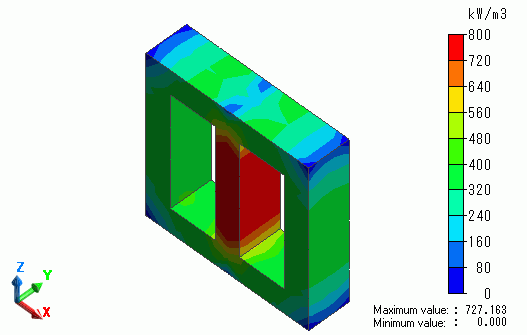The temperature contour as a result of Watt is shown below.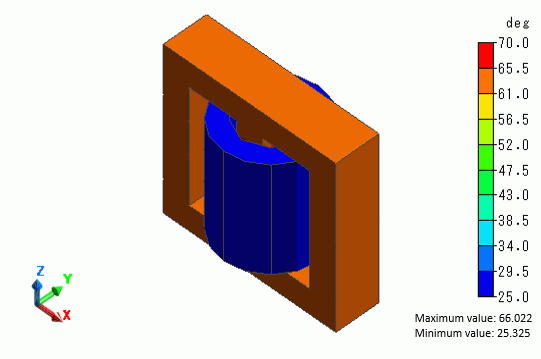The core temperature goes up to around 66[deg].

﻿